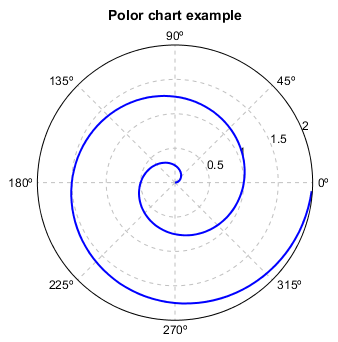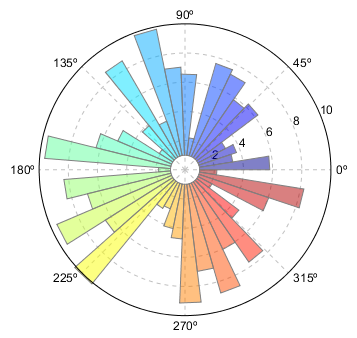# Polar plot¶

Polar axes can be created using `axes(polar=True)` function. Then use `plot`, `bar` and other plot functions to plot the data in the polar axes.

Plot a line in a polar axes

```r = arange(0, 2, 0.01)
theta = 2 * pi * r

ax = axes(position=[0.1,0.1,0.8,0.8], polar=True)
plot(theta, r, color='b', linewidth=2)
title('Polor chart example')
antialias(True)
```Plot bars in a polar axes

```# Compute pie slices
N = 40
theta = linspace(0.0, 2 * pi, N, endpoint=False)